## Abstract

Piezoelectric Macro-Fiber Composite (MFC) utilization is increasing in engineering fields due to its strong actuation forces and high flexibility. In this paper, piezoelectric (${\textstyle {d}_{33}MFC}$) type (M8528-P1) patches are applied for active control of a cantilever composite beam. A linear coupled ﬁnite element model for piezoelectric MFC actuation of the composite beam was developed based on the APDL-ANSYS codes by using linear piezoelectric constitutive equations to study smart composite beam behavior in open and closed-loop cases. Active vibration control response of laminated composite beams with various stacking sequence configurations was examined, and the results were compared. In this, the proportional type ($kp$) of control algorithms is utilized, and in the summary of work refined two sets of finite element models. The first set their laminates to have an orientation (0 with 90), and others have an orientation (0 with 45). When the control signal applied with the gain ($kp$) to a system increases, the rise time generally decreases, the actuator voltages for different control gain for all cases are observed with the increase in the proportional constant. The findings of this study indicate that the composite beams composed from (0/90)stacking sequence sets had reached stability state faster than the composite beams composed from (0/45) stacking sequence sets.

Keywords: MFC piezoelectric, smart composite beam, FEM (APDL Codes), active vibration

## 1. Introduction

Vibration is present in countless daily life applications, and more often, vibration is highly undesirable. Undesirable vibration may decrease the performance of the product and cause safety or economic issues . Vibration damping includes active, passive, and hybrid damping techniques. It is important to understand that passive damping can be very efficient in damping out high-frequency excitation, while active damping can be used to control low-frequency vibrations . Active vibration-reducing techniques turn out to be a reality for a wide variety of applications . An active method integrates sensors and actuators with a flexible structure, operated by a control scheme. An essential issue in active control systems is sensor and actuator selection . The ever-increasing use of smart composite materials in advanced areas such as aerospace, automotive, and sports equipment has motivated researchers to explore behavior and performance characteristics .

There are some previous studies reported in this work. The simple cantilever beams active vibration control is investigated in [6-10]. Piezoelectric patches are mounted on the beams as actuators. With the purpose of sensing the vibration level, a strain-gage or another piezoelectric patch can be used. The identification system and placement control technique was utilized in . The piezo patches and beam structure’s finite element model is constructed, and the closed-loop control is applied in [7,8]. Also, utilized the model of the beam and piezo-patches finite element; however, modal control techniques were employed Singh et al. . Xu and Koko used the commercial finite element package ANSYS to state the findings . The control design was conducted in the space state that was set in the analysis of the finite element model. For the control design, MATLAB as a system toolbox was utilized in their experiment. The sensors/actuators influence site was investigated. It was noticed that the site near the fixed end for the vibration control was better. Lim investigated several modes for vibration control of a fixed square plate by using devices of location discrete sensor/actuator at maximum strain points . Quek et al. introduced a typical placement method of pairs of piezoelectric sensor /actuator for the vibration control sealed compound plates . Xiamin et al. used four-bar linkage to investigate active vibration control . The model of the finite element is applied and the reduced mode, robust ${\textstyle {H}_{\infty }}$, and standard H∞ control methods are analyzed. Halim and Moheimani investigated a set of proposals to a criterion for ideal placement of a collected piezoelectric sensor-actuator pair on their supported plate by using modal and locative controllability . Bendine et al. studied the modeling of the active vibration control of a smart functionally graded (FGM) beam based on a higher-order shear deformation theory . The locations of piezoelectric actuators and sensors have a great influence on the reliability and control system efficiency. Malgaca studied optimal parameters with respect to positive position feedback used in the real-time control algorithm determined with the objective functions . Flexible single-link composite manipulator with [45/-45] and [0/90] lay-ups are performed to reduce endpoint vibrations. Takacs and Rohal-Ilkiv  proposed a direct closed-loop prototype method in the active vibration control system modeled in the finite element method using APDL language for the clamped-free aluminum beam piezoelectric actuation using a linear quadratic controller (LQ) with a Kalman-ﬁlter. Vashist and Chhabra  found the optimal piezoelectric actuator’s locations by using an integer coded genetic algorithm GA on their plate where the singular value decomposition’s control matrix was used as a fitness function. Similar results of the frequency responses between experimental and Finite Element Method were found. Yavuz et al. analyzed a system of active vibration control’s closed-loop with the purpose of suppressing the vibrations of the end-point . They established the system’s mathematical model by using the Lagrange equations. The digital solution is achieved through mixing the Newmark technique with the control action. They applied PID control action with the purpose of finding the value of the actuator signal at the appropriate time. Liu X. et al. studied the piezoelectric actuators’ placement optimization and the membrane structure’s active vibration control . They designed the classical linear quadratic regulator controllers with the aim of suppressing the undesired vibration. Simulation findings indicated that the piezoelectric actuators’ optimal locations are influenced by the actuator’s additional mass and stiffness. Van et al. developed a finite element model according to the theory of first-order shear deformation for active vibration control and optimal placement of laminated composite plates with linked pairs of distributed piezoelectric sensor/actuator . Baghaee et al. developed the aero elastic panel flutter of the laminate composite plate to include two piezoelectric (MFC-type) . They determined MFC actuator’s input voltage through the velocity or proportional feedback control algorithms according to the sensor output. Usman et al. used piezoelectric MFC energy harvesting on the cantilever beam connected to a downstream cylinder and placed stationary upstream cylinder before applying wind flow on both cylinders . Optimum spacing between two cylinders was identified as being three times the circular cylinder diameter. Heganna and Joglekar  studied the forced vibration control analysis of the beam structure experimentally. The smart structure considered ﬁnite and ﬂat cantilever beam-like ﬁber structure with piezoelectric PZT patches attached on the surface.They also analyzed the vibration control effect of adjusting the location of PZT patches. Xie et al. proposed the fractional-order PD$\mu$ algorithm on the active vibration control of a lattice grid beam . The vibration responses and dynamic model was based on the third-order shear deformation theory. A new active vibration control method is implemented using the PD$\mu$ algorithm in fractional order. Rimašauskienė et al. developed and experimentally analyzed the passive and active vibration control techniques for a thin-walled composite beam . After the experiments with active vibration control, it was noticed that the vibration damping of the analyzed system was far higher at its resonant frequency in all cases. However, it could be inferred from the experimental results that passive and active vibration control systems were effective for thin-walled composite beam vibration amplitude control.

This work deals with the active vibration control analysis in smart composite beam structures by using ANSYS APDL codes. All nodes in the composite structure beam are subjected to the boundary condition (exactly 1260 boundary condition), and different proportional control gain is used to control vibration suppression to two sets of the composite beams, (0/90) sets and (0/45) sets, to reach the steady-state. From the obtained results, it can be concluded that the technique is able to dump the vibration of the composite beam structure.

## 2. Piezoelectric constitutive equations

Under the influence of stresses and voltage, the magnitude of the strain result and amount of electric charge accumulated by piezoelectric material specifies the actuation and sensing characteristics of these materials. Both constituent relationships under a piezoelectric material may be used to quantify these properties. Macroscopically, the piezoelectric materials display a field–strain relation . The relationship is almost linear for the low electrical field, which can provide many advantages when using piezoelectric materials for system modeling and control. Nevertheless, the polarization saturates in a high electrical field, and domains extend and switch. This induces significant non-linear behavior that may be detrimental to the use of piezoelectric materials In the control implementation associated with a high electrical field . In several applications of piezoelectric materials to continuous structures, the linear behavior is adopted despite the nonlinear behavior at a high electric field. The direct and converse effect of the piezoelectric phenomena involves an interaction between the mechanical behaviors of a material; Linear constitutive equations contain two mechanical variables and two electrical variables . The equations governing the direct and converse piezoelectric effect in the matrix form are expressed respectively as

 $\left\{D\right\}={[e]}^{T}\,\left\{S\right\}+\left[{\epsilon }^{S}\right]\left\{E\right\}$
(1)
 $\left\{T\right\}={[c}^{E}]\,\left\{S\right\}+\left[e\right]\left\{E\right\}$
(2)

where ${\textstyle \left\{D\right\}}$ is the electric displacement, ${\textstyle {[e]}^{T}}$ is the transpose of the dielectric permittivity matrix ${\textstyle \left[e\right]}$, ${\textstyle \left\{S\right\}}$ is the mechanical strain vector, ${\textstyle \left[{\epsilon }^{S}\right]}$ is the dielectric permittivity matrix at constant mechanical strain, ${\textstyle \left\{E\right\}\,}$ is the electric intensity vector, ${\textstyle \left\{T\right\}}$ is the mechanical stress vector and ${\textstyle {[c}^{E}]}$ is the matrix of elastic stiffness at constant electric field strength. The same relationship can be written in three other forms, depending on which variables are chosen to be independent. The direct relationship given by Eq.1 is normally used when modeling the sensing capability of the piezoelectric material, while the actuator capability is modeled using the converse effect relationship given by Eq. (2) .

## 3. Simulation method

In this section, ANSYS software simulates active vibration control in the smart composite. Figure 1 shows the block diagram of the obtained analysis. ANSYS/Multiphysics and ANSYS/Mechanical programs can be used for modeling structural fields with piezoelectric smart patches, whereas ${\textstyle {F}_{e}\,}$ is the force of vibration generated. The instant value of vibration producing force ${\textstyle {F}_{e}}$ can be described at each time step. In the analysis below, it is taken as ${\textstyle {F}_{o}}$ at ${\textstyle t\,=\Delta t}$ and zero for other time steps. At a piezoelectric sensor location, the strain ($\varepsilon$) is calculated. Reference input for vibration cancellation is zero. ${\textstyle \,{K}_{p}}$, ${\textstyle {K}_{v}}$, and ${\textstyle {K}_{s}}$ are respectively, the variables the control, power, and sensor amplification factors. ${\textstyle {K}_{v}\,}$ is taken as 200, and ${\textstyle {K}_{p}\,}$ is changed in the analysis below. Only the proportional control is applied. The proportional constant for the actuator voltage ${\textstyle {V}_{a}}$ was obtained from the multiplication of ${\textstyle {K}_{p}{\,K}_{v}\,{K}_{s}}$. Therefore, the results are not affected by changing the values of ${\textstyle {K}_{s}}$, ${\textstyle {K}_{p}}$ and ${\textstyle {K}_{v}}$ and keeping their multiplication the same. To evaluate the performance of the vibration control, the calculated deflection at a location, ${\textstyle dt}$, is observed.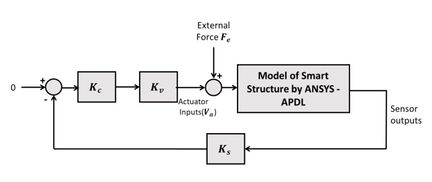Figure 1. Block diagram of the analysis

First, the structures of the beam type are considered. The configuration of the beam composite structure is shown in Figure 2.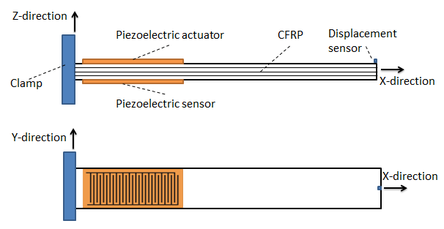Figure 2. Configuration for the structure of the beam type

#### 3.1 Material Properties

Finite Element Analysis is used as the numerical modeling tool to simulate the dynamic characteristics of the composite structures by ANSYS macro codes, and the macro file has been written by using APDL. The first step in the coding process defined model geometry by using direct mesh generation of smart structure. In first our system, we used a composite smart beam of size (400 mm $\times$ 28 mm ) and various thickness (1.59 mm, 2.12mm and 3.18mm) according to number of laminates, with (${\textstyle {d}_{33}MFC-}$$P1$) piezoelectric patch actuator type (M8528-P1). These typically consist of an active area (85mm $\times$ 28 mm) with (0.3 mm) thickness, and it is embedded on the beam from (10 mm) on the fixed point, as shown in Table 1 for all cases.

#### 3.2 Simulation procedures

In the first step, the beam structures type is taken and considered. Figure 2 displays the configuration of the structure with sensor locations. The displacement value is taken from the displacement sensor, and similarly, the strain value is taken from the strain sensor as the feedback. Here, the product of ANSYS /Multiphysics (version 18) was applied to the structures of the smart composites model. The combination of the ANSYS modeling and solution into the control action codes were achieved by ANSYS .

The composite smart structure beam is studied, as indicated in Table 1.

Table 1. Dimensions and distances of Composite beam structure
 Case No. Composite layers Dimensions of the structure (mm) 1 (0/90/0) $400\times 28\times 1.59$ 2 (0/90/0/90) $400\times 28\times 2.12$ 3 (0/90/90/0) $400\times 28\times 2.12$ 4 (0/90/0/0/90/0) $400\times 28\times 3.18$ 5 (0/45/0) $400\times 28\times 1.59$ 6 (0/45/0/45) $400\times 28\times 2.12$ 7 (0/45/45/0) $400\times 28\times 2.12$ 8 (0/45/0/0/45/0) $400\times 28\times 3.18$

The macro file creates nodes and finite elements to the structure. In the first part, composite materials have used element type SOLID185 layered structural elements for composite beam or plate part, and element type (SOLID5) elements with (MASS21) were used in the second structure part for the piezoelectric part in the structure . Overall, these macro codes as shown in Table 2 indicate the defined element types of various materials.

Table 2. Element definition codes
 /PREP7 ! start the preprocessing ! Define Element type #1 ET,1,185 ! chooses SOLID185 element for analysis composite material KEYOPT,1,3,1 ! Key option #3 = 1 LAYERED SOLID ELEMENTS KEYOPT,1,2,2 ! Key option #2 = 2 ENHANCED STRAIN FORMULATION KEYOPT,1,8,1 ! Key option #8 = 1 STORE DATA FOR ALL LAYERS ! Define Element type #2 ET,2,solid5,3 ! chooses SOLID5 element for analysis piezoelectric material ET,3,mass21

Therefore, an essential next step to confirming in three-dimensional composite material properties is assigned. As shown in Figure 2, the structure is divided into two parts. The first part deals with composite material, and the other part is actuator and all properties, as shown in Table 3, and all that has been gained from [32,33,34].

Table 3. Material properties of MFC and CFRP
 Material Properties CFRP MFC Young’s modulus ${\textstyle {\boldsymbol {E}}_{11}}$ 140 GPa 30.34 GPa Young’s modulus ${\textstyle {\boldsymbol {E}}_{22}}$ l8.75 GPa 15.86 GPa Young’s modulus ${\textstyle {\boldsymbol {E}}_{33}}$ 8.75 GPa 9.03 GPa Shear modulus ${\textstyle {\boldsymbol {G}}_{12}}$ 7.2 GPa 5.52 GPa Poisson’s ratio ${\textstyle {\boldsymbol {v}}_{12}}$ 0.3 0.31 Poisson’s ratio ${\textstyle {\boldsymbol {v}}_{13}}$ 0.3 0.30 Density $\rho$ 1550 kg/m$^{3}$ 5020 kg/m$^{3}$ Piezoelectric Stress constant (${\textstyle {\boldsymbol {e}}_{32}}$) -3.47 C/m$^{2}$ Piezoelectric Stress constant (${\textstyle {\boldsymbol {e}}_{33}}$) 12.36 C/m$^{2}$

The ANSYS macro of the material properties is given in Table 4. The first material is represented by composite material (Carbon fiber reinforced polymer), and the second material is represented by the piezoelectric (MFC) actuator material.

Table 4. Composite material properties codes
 /PREP7 ! Define Material 1# : ${\textstyle E1=140\times {10}^{9}\,Pa}$ MP,EX,1,140E9 MP,EY,1,8.75E9 ! Define Material 1# : ${\textstyle E2=8.75\times {10}^{9}\,Pa}$ MP,EZ,1,8.75E9 ! Define Material 1# : ${\textstyle EZ=EY\,;\,E3=8.75\times {10}^{9}\,Pa}$ MP,GXY,1,7.2E9 ! Define Material 1# : shear modulus xy-plane ${\textstyle {G}_{xy}=}$$7.2\times {10}^{9}\,Pa$ MP,GYZ,1,6.21E9 ! Define Material 1# : shear modulus xz-plane ${\textstyle {G}_{xy}=6.2\times {10}^{9}\,Pa}$ MP,GXZ,1,7.2E9 ! Define Material 1# : shear modulus yz-plane ${\textstyle {G}_{xy}=7.2\times {10}^{9}\,Pa}$ MP,PRXY,1,0.3 ! Define Material 1# : Poisson coefficient ${\textstyle {\upsilon }_{1}=0.3}$ MP,PRYZ,1,0.3 ! Define Material 1# : Poisson coefficient ${\textstyle {\upsilon }_{2}=0.3}$ MP,PRXZ,1,0.3 ! Define Material 1# : Poisson coefficient ${\textstyle {\upsilon }_{3}=0.3}$ MP,DENS,1,1550 ! Define Material 1# : Density for composite material

The number of composite layers, the thickness of layers, and orientation angles of fibers are assigned as macro APDL ANSYS codes for the first material, as given below in Table 5.

Table 5. Orientation angles’ Composite layers codes
 SECTYPE,1,SHELL set #1, [0/90/0] SECDATA,0.53e-3,1,0 ! 1st LAYER 1: Thk=0.53e-3m, THETA = 0° SECDATA,0.53e-3,1,90 ! 2nd LAYER 2: Thk=0.53e-3m, THETA = 90° SECDATA,0.53e-3,1,0 ! 3rd LAYER 4: Thk=0.53e-3m, THETA = 0°

The second material piezoelectric actuator properties are given in Table 6 as macro ANSYS.

Table 6. MFC properties codes
 ! Define dielectric constant MP,DENS,3,5020 ! Density for MFC piezoelectric material MP,PERX,3,15.586E-9 ! Permittivity in X direction MP,PERY,3,15.586E-9 ! Permittivity in Y direction MP,PERZ,3,11.79E-9 ! Permittivity in Z direction TB,PIEZ,3 ! Define piezoelectric table TBDATA,16,0 ! e16 piezoelectric constant TBDATA,14,0 ! e25 piezoelectric constant TBDATA,6,-3.47 ! e32 piezoelectric constant TBDATA,9,12.36 ! e33 piezoelectric constant TB,ANEL,3 ! Define structural table

After all dimensions and properties of both composite and piezoelectric properties are defined, the type of element is assigned for each type of material. SOLID185 layered structural elements are assigned for the composite part, and SOLID5 elements are used for the piezoelectric part of the structure. Then, the static solution is analyzed to evaluate the initial deflections of each node and element. The model is discretized into finite elements model, as shown in Figure 3.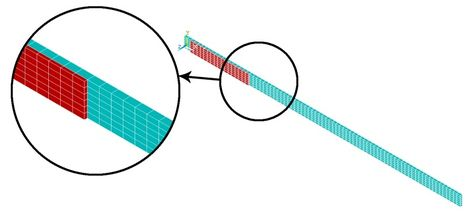Figure 3. Finite element model

The first three mode shapes and natural frequencies of the composite plates were obtained using APDL ANSYS macro codes. The obtained results were shown in Figure 4. The first three natural frequencies evaluated by the modal shape analysis method using ANSYS (15.665 Hz), (94.17Hz), and (161.63Hz), respectively, for the first case considered in the present study, are shown in Figure 4.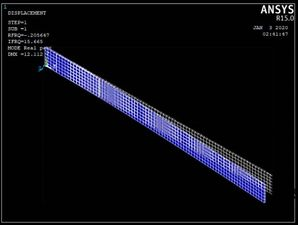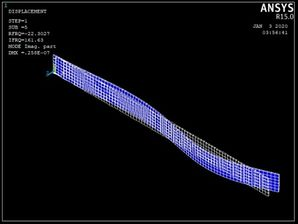1st mode shape 2nd mode shape 3rd mode shape Figure 4. Mode shapes of the first case

Fixed boundary conditions are described for the nodes ${\textstyle \,(x=0)}$ and given in Table 7 as macro ANSYS.

Table 7. Boundary conditions of composite beam codes
 CP,1,volt,all ! set #1 of coupled degrees of freedom.

The degrees of freedom of the top and bottom surfaces for piezo actuator and sensor are coupled simultaneously with volt by APDL ANSYS “CP” command. Modal analysis was performed to determine parameters from vibration data measures like the inherent dynamic characteristics of the system in forms of natural frequencies, mode shapes and damping factor, and so to determine time step .

Householder method (reduced method) can be used for the beam structures which have the solid coupled field in ANSYS. The time step is the incremental change in time and the time step is chosen by equation formula ${\textstyle \Delta t\,=1/(20\,{f}_{h})}$, where ${\textstyle {f}_{h}\,}$ is the highest natural frequency to be considered. Table 8 shows the three natural frequencies for the undamped system. The first mode is considered to calculate the time step and ${\textstyle \Delta t}$ is 0.0032, 0.0034, 0.0025, 0.00183, 0.00318, 0.0032, 0.0025, and 0.0018, for cases 1, 2, 3, 4, 5, 6, 7, and 8, respectively.

In this study, The strain feedback (piezoelectric sensor) is calculated at the selected sensor location, multiplied by ${\textstyle {Ks}_{1}}$ to convert the strain feedback to voltage and then subtracted from zero to calculate the error signal.

The displacement feedback is also calculated at the selected sensor location, multiplied by ${\textstyle {Ks}_{2}}$ in order to convert the displacement feedback to voltage, and also subtracted from zero to evaluate the error signal between the input and output.

Table 8. Natural frequencies of the system
 Case No. Composite layers First (Hz) Second (Hz) Third (Hz) 1 (0/90/0) 15.665 94.17 161.63 2 (0/90/0/90) 14.888 87.674 193.47 3 (0/90/90/0) 19.795 120.39 196.81 4 (0/90/0/0/90/0) 27.265 165.45 217.71 5 (0/45/0) 15.701 94.379 170.62 6 (0/45/0/45) 15.589 92.137 200.82 7 (0/45/45/0) 19.945 121.29 204.18 8 (0/45/0/0/45/0) 27.697 168.02 222.82

The difference between the input reference and the sensor signal is defined as the error signal. The error value is multiplied by ${\textstyle {Kp}_{1}}$ for strain feedback and ${\textstyle Kp\,}$ for displacement feedback, and then both results are multiplied by the amplifier factor ${\textstyle Kv}$ to determine ${\textstyle Va}$ at a given time step.

The macro part that enables the calculations for the analysis of the closed loop for ${\textstyle t>\Delta t}$ is given in Table 9.

Table 9. Sensor feedback codes
 *do,tt,2*dt,ts,dt !time loops *get,uz-tip,node,nldm,u,z !sensor feedback err=ref-ks*uz-tip !error signal Vact=kp*kv*err !actuator voltage d,nv,volt,Vact !Appling actuator volt time,tt solve *enddo

## 4. Results and discussion

There are two methods to deal with active vibration control. The first method is displacement feedback signals by using the displacement sensor, and the other method is strain feedback signals by using a piezoelectric strain sensor. Displacement feedback signals have been used for the displacement to suppression of vibration.

Classical controller ($P$) is the proportional control and gain ($kp$) is the only variable to be changed. The present study has been used for (2,4,6, and 8) $kp$ value. The open-loop and closed-loop responses of the tip displacement are shown in Figures 5(a), (b), (c) and (d), respectively, by using APDL ANSYS. From the graph, it is found that the open-loop response vibrates a lot at a time of more than 5 sec in first Figure 5(a), 4 sec in second Figure 5(b), 3.8 in third Figure 5(c), and 1.25 sec in the last Figure 5(d) . In the first case, composite laminates consist of three-ply ($0/90/0$) closed-loop responses by using classical controller when we took into consideration only proportional part effects in PID control, which is shown settle at a time 1.4 sec, 0.85 sec, 0.75 sec and 0.7 sec, respectively, proportional gain ($kp$) is 2, 3, 6, 8, which demonstrates that three recent responses of the system will be near each other. This reduction in the time compared with the first case is due to the increase in the number of composite layers, and, at the same time, an increase in the strength properties of the beam is observed.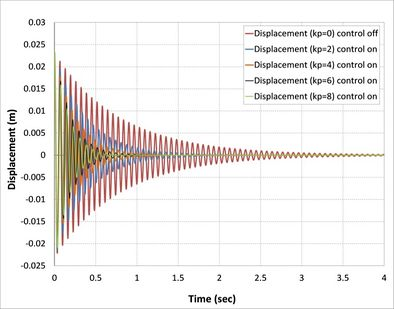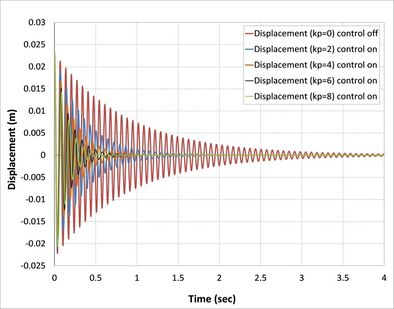(a) CFRP (0/90/0) (b) CFRP (0/90/0/90)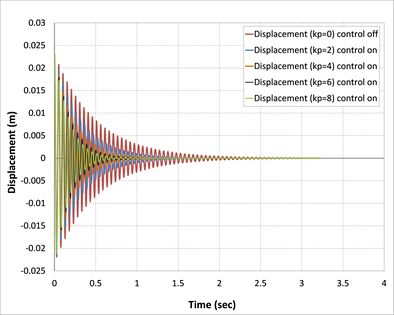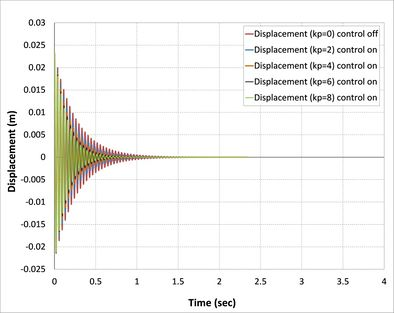(c) CFRP (0/90/90/0) (d) CFRP (0/90/0/90/0/90 Figure 5. Open-loop and closed-loop responses of a composite beam of tip displacement

However, the evidence for this relationship is conclusive and has been retrieved from PID rules; with increasing the proportional range, the vibration converges slowly and settling time increases. On the other side, the controlling signal has been decreasing by increasing the value of the proportional constant that takes part in PID and neglected integral and derivative parts. Whereas in the second case, composite laminates consist of four plies (0/90/0/90), the closed-loop responses or all proportional gain ($kp$) shown as in Figure 5(b) is close to the results of the first case because the effect of the fourth ply is smaller (90 degree) composite ply with the beam in the fourth case, which demonstrates that control responses of the system will be near each other and this reduction in the time compared with others is due to the increase in the number of composite layers and at the same time increases the strength properties of the beam. Based on the results, it has been noted that an increase in laminates of composite plate decreases the vibration and time stability of the system.

For amplitude comparison between two sets of composite materials beam structures, first set consist of (0-90) degrees of laminates and another set consist of (0-45) degrees of laminates. This based on the responses that were simulated with high accuracy using the proportional gain formulation. As shown in Figures 5 and 6, all curves have nearly the same shape, but speed to reach the stable case in the second set is smaller than the first set. The reason is attributed to the greater flexural strength for the second compared to the former.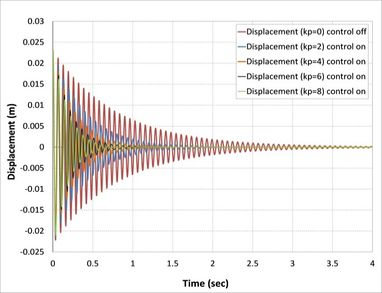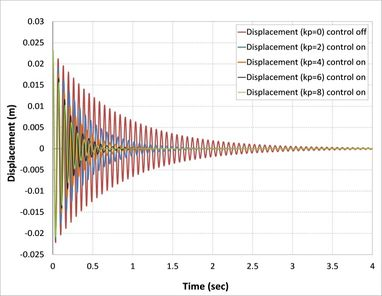(a) CFRP (0/45/0) (b) CFRP (0/45/0/45)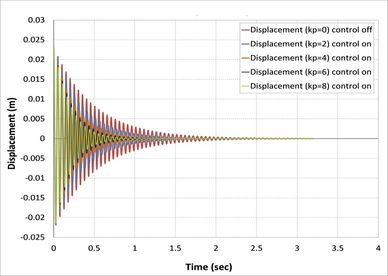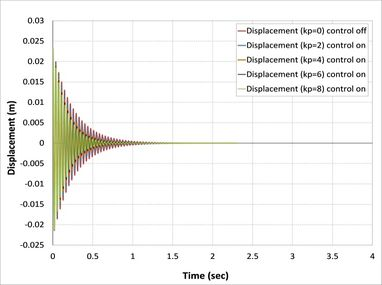(c) CFRP (0/45/45/0) (d) CFRP (0/45/0/45/0/45) Figure 6. Open-loop and closed-loop responses of the composite beam of tip displacement

Piezoelectric MFC has a maximum voltage, so it is peak to peak voltage. While peak voltage is the voltage from the zero horizontal axis line to the top of the waveform, the peak to peak voltage is the voltage of the one cycle of a wave, all the way from the peak of the negative side to the peak of the positive side . The maximum voltage for MFC to be applied is (2000 volt) for the piezoelectric actuator. The uncontrolled and controlled responses obtained by actuation voltage can be seen in Figures 7(a), (b), (c), (d), (e), (f), (g), and (h). From the graph, it has been observed that in the open-loop, the response was nil because in the open-loop, there is no link between vibrate response and actuator.

On the other hand, in all cases, when the control signal applied with the gain to a system increases, the rise time generally decreases as shown in Figure 7, where the actuator voltages for different control gain for all cases are observed to increase with a proportional constant. Meanwhile, $kp=8$ has the highest value of actuator voltage observed in all cases .

As can be seen from Figures 7(b), (c), (d), (f), (g), and (h), the minimum value of actuator voltage is (-500V) and smaller than this volt by APDL-ANSYS macro file has been changed to (-500 Volt) because the sinusoidal voltage of piezoelectric MFC-P1 is worked between (-500 volt) to (+1500 Volt) voltage cycle at 0.1 Hz was output by a voltage amplifier .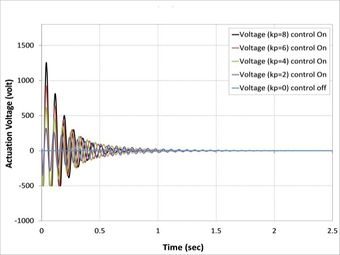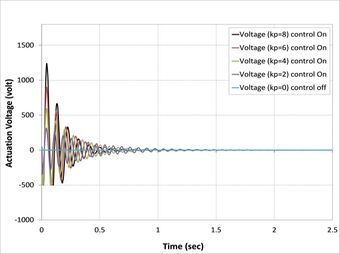(a) CFRP (0/90/0) (b) CFRP (0/90/0/90)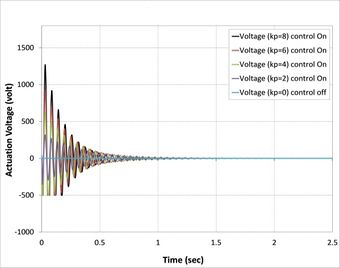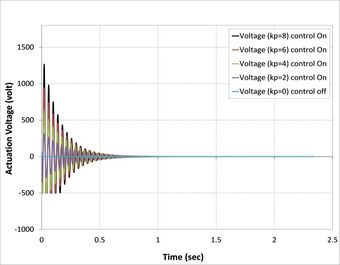(c) CFRP (0/90/90/0) (d) CFRP (0/90/0/0/90/0)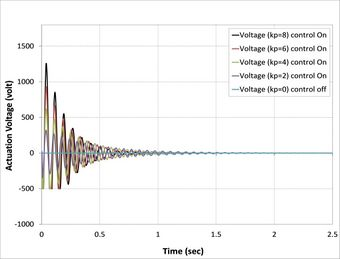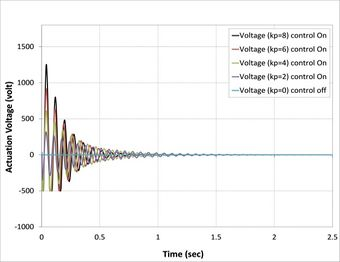(e) CFRP (0/45/0) (f) CFRP (0/45/0/45)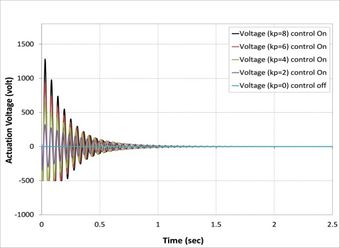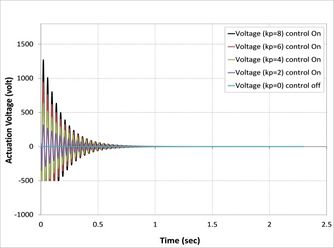(g) CFRP (0/45/45/0) (h) CFRP (0/45/0/0/45/0) Figure 7. Open-loop and closed-loop responses of the composite beam of the actuation voltage

Another important finding was the effect of fiber orientations of composite laminate layers on active vibrations, as shown in Figure 8. The composite beams composed of laminates (0/90/0), (0/90/0/90), (0/90/90/0), and (0/90/0/0/90/0) reach stability faster than the composite beams composed of laminates (0/45/0), (0/45/0/45), (0/45/45/0), and (0/45/0/0/45/0) because the first set has higher relaxation modulus than second sets .

## 5. Conclusions

A FEM for piezoelectric with composite beam structure was proposed to simulate model dynamic, and the electrical model of piezoelectric which permitted the simulations of type (${\textstyle {d}_{33}-}$$MFC$) effects. The performance of the composite beam structure is improved when piezoelectric type M8528(P1) is used. It is shown that the classic controller provides in increasing control gain (${\textstyle kp}$) and better active damping than low control gain, especially at ${\textstyle kp=8}$.

The active control capability of such a composite beam is analyzed using a simple control strategy. Overall, these controlled responses show that, although all different composite laminate plates are subjected to the same intensity of mechanical load. The observed decrease in the vibration of the composite plate might be explained by the increase in the proportional gain in PID control on all cases of the composite laminate plate, while at the same time decreasing the voltage actuator of piezoelectric. The model can be used for more investigations with general composition, applied loads, and another control method. The final objectives of analysis and modeling are the minimization of weight (or mass) of the beam structure under behavior constraints, increasing the performance of the piezoelectric actuator, and maximization of natural frequencies of the vibration modes. However, given the immersed short time for each case, caution must be taken. The difference between two similar different in orientation laminate, two refined finite element models based 0/90 laminate set and 0/45 laminate set, for the analysis of laminated beam with embedded surface bonded piezoelectric laminae have been presented. The evidence from this study suggests the composite beams composed from (0/90) stacking sequence sets had reached stability state faster than the composite beams composed from (0/45) stacking sequencesets.

### Document informationPublished on 22/04/20
Accepted on 13/04/20
Submitted on 21/02/20

Volume 36, Issue 2, 2020
DOI: 10.23967/j.rimni.2020.04.001

### Document Score0

Views 256
Recommendations 0